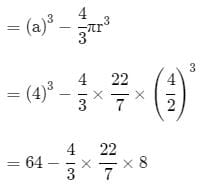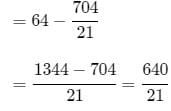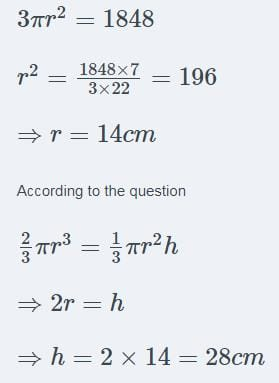Test: Surface Area & Volumes- 2

# Test: Surface Area & Volumes- 2

Test Description

## 25 Questions MCQ Test Mathematics (Maths) Class 9 | Test: Surface Area & Volumes- 2

Test: Surface Area & Volumes- 2 for Class 9 2022 is part of Mathematics (Maths) Class 9 preparation. The Test: Surface Area & Volumes- 2 questions and answers have been prepared according to the Class 9 exam syllabus.The Test: Surface Area & Volumes- 2 MCQs are made for Class 9 2022 Exam. Find important definitions, questions, notes, meanings, examples, exercises, MCQs and online tests for Test: Surface Area & Volumes- 2 below.
Solutions of Test: Surface Area & Volumes- 2 questions in English are available as part of our Mathematics (Maths) Class 9 for Class 9 & Test: Surface Area & Volumes- 2 solutions in Hindi for Mathematics (Maths) Class 9 course. Download more important topics, notes, lectures and mock test series for Class 9 Exam by signing up for free. Attempt Test: Surface Area & Volumes- 2 | 25 questions in 25 minutes | Mock test for Class 9 preparation | Free important questions MCQ to study Mathematics (Maths) Class 9 for Class 9 Exam | Download free PDF with solutions
 1 Crore+ students have signed up on EduRev. Have you?
Test: Surface Area & Volumes- 2 - Question 1

### The total surface area of a cube is 96 cm2 . The volume of the cube is

Detailed Solution for Test: Surface Area & Volumes- 2 - Question 1

Surface area of a cube = 96 cm2
Surface area of a cube = 6 (Side)2 = 96 ⇒  (Side)2 = 16
⇒ (Side) = 4 cm
[taking positive square root because side is always a positive quantity]
Volume of cube = (Side)3 = (4)3 = 64 cm3
Hence, the volume of the cube is 64 cm3.

Test: Surface Area & Volumes- 2 - Question 2

### If the outer diameter of a pipe 21 m long is 1 m, then its curved surface area is

Test: Surface Area & Volumes- 2 - Question 3

### A cube of side 4 cm contains a sphere touching its sides. Find the approximate volume of the gap in between.

Detailed Solution for Test: Surface Area & Volumes- 2 - Question 3

Let ABCD be cube which contain sphere touching its sides.The diameter of sphere is the sides of the cube.

Now,

The volume of the gap in between cube and sphere

= volume of cube - volume of sphere\

= 30.48 cm3

Test: Surface Area & Volumes- 2 - Question 4

The cost of digging a pit of dimensions 4.5m×2.5m×2.5m at the rate of Rs 20 per cubic metre is

Test: Surface Area & Volumes- 2 - Question 5

If the volume of a sphere is 4851 cm3, then its surface area is

Test: Surface Area & Volumes- 2 - Question 6

The lateral surface area of a cube is 256 m2 The volume of the cube is

Test: Surface Area & Volumes- 2 - Question 7

The cost of cementing the inner curved surface of a 14 m deep well of radius 2 m at the rate of Rs 2 per m2 is

Detailed Solution for Test: Surface Area & Volumes- 2 - Question 7

Depth of well(h) = 14m
radius of well(r) = 2m

Inner surface area of well(like a cylinder) = 2πrh

surface area= 2 x 22/7 x 2 x 14 = 176 m2

cost of cementing = ₹2 per m2

Total Cost = 176 x 2 = ₹352

Test: Surface Area & Volumes- 2 - Question 8

The ratio of the radii of two spheres whose volumes are in the ratio 64 : 27 is

Test: Surface Area & Volumes- 2 - Question 9

The volume of resulting cuboid formed when two cubes each of side 6 cm are joined end to end is

Test: Surface Area & Volumes- 2 - Question 10

A hemispherical bowl is made of steel 0.25 cm thick. If the inner radius of the bowl is 3.25 cm, then the outer curved surface area of the bowl is

Test: Surface Area & Volumes- 2 - Question 11

The difference between the total surface area of a cube of side 4 cm and its lateral surface area is

Test: Surface Area & Volumes- 2 - Question 12

The diameter of the base of a cylinder of curved surface area 88 cm2 and height 14 cm is

Test: Surface Area & Volumes- 2 - Question 13

Given that the surface area of a spherical shot put is 616 cm2. its diameter is

Test: Surface Area & Volumes- 2 - Question 14

The number of litres that a cuboidal water tank of dimensions 6m×5m×4.5m can hold is

Test: Surface Area & Volumes- 2 - Question 15

If the surface area of a solid sphere is 1386 cm2. then the total surface area of the solid hemisphere of the same radius is

Test: Surface Area & Volumes- 2 - Question 16

The volume of a cube whose diagonal is 8√3 cm is

Test: Surface Area & Volumes- 2 - Question 17

The total surface area of a right circular cylinder of height 4 cm and radius 3 cm is

Test: Surface Area & Volumes- 2 - Question 18

If a spherical balloon grows to twice its radius when inflated, then the ratio of the volume of the inflated balloon to the original balloon is

Test: Surface Area & Volumes- 2 - Question 19

The surface area of a cuboid whose length, breadth and height are 15 cm, 10 cm and 20 cm respectively is

Test: Surface Area & Volumes- 2 - Question 20

The number of spherical bullets each 5 dm in diameter which can be cast from a rectangular block of lead 11 m long, 10 m broad and 5 high is

Test: Surface Area & Volumes- 2 - Question 21

The number of planks of dimensions (5m×25cm×10cm) that can be placed in a pit which is 20 m long, 6 m wide and 80 cm deep is

Test: Surface Area & Volumes- 2 - Question 22

If the lateral surface area of a cylinder is 132 cm2 and its height is 7 cm, then its base diameter is

Test: Surface Area & Volumes- 2 - Question 23

If the total surface area of a hemisphere is 1848 cm2, then the diameter is

Detailed Solution for Test: Surface Area & Volumes- 2 - Question 23Test: Surface Area & Volumes- 2 - Question 24

The volume of a cylinder whose circumference of the base is 132 cm and height 25 cm is

Test: Surface Area & Volumes- 2 - Question 25

The number of solid sphere each 6 cm in diameter, which can be moulded from a solid cylinder of height 45 cm and diameter 4 cm without any loss is

## Mathematics (Maths) Class 9

84 videos|352 docs|109 tests
 Use Code STAYHOME200 and get INR 200 additional OFF Use Coupon Code
Information about Test: Surface Area & Volumes- 2 Page
In this test you can find the Exam questions for Test: Surface Area & Volumes- 2 solved & explained in the simplest way possible. Besides giving Questions and answers for Test: Surface Area & Volumes- 2, EduRev gives you an ample number of Online tests for practice

## Mathematics (Maths) Class 9

84 videos|352 docs|109 tests